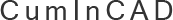CumInCAD is a Cumulative Index about publications in Computer Aided Architectural Design supported by the sibling associations ACADIA, CAADRIA, eCAADe, SIGraDi, ASCAAD and CAAD futures
 PDF papersReferences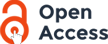authors Nadler, Edmond 1985 Piecewise Linear Approximation on Triangulations of a Planar Region Reports in Pattern Analysis. , V, 76 p. :ill. May, 1985. No. 140. includes bibliography For any triangulation of a given polygonal region, consider the piecewise linear least squares approximation of a given smooth function u. The problem is to characterize triangulations for which the global error of approximation is minimized for the number of triangles. The analogous problem in one dimension has been thoroughly analyzed, but in higher dimensions one has also to consider the shapes of the subregions, and not only their relative size. After establishing the existence of such an optimal triangulation, the local problem of best triangle shape is considered. Using an expression for the error of approximation involving the matrix H of second derivatives, the best shaped triangle is seen to be an equilateral transformed by a matrix related to H. This triangle is long in the direction of minimum curvature and narrow in the direction of maximum curvature, as one would expect. For the global problem, a series of two lower bounds on the approximation error are obtained, which suggest an asymptotic error estimate for optimal triangulation. The error estimate is shown to hold, and the conditions for attaining the lower bounds characterize the sizes and shapes of the triangles in the optimal triangulation. The shapes are seen to approach the optimal shapes described in the local analysis, and the errors on the triangles are seen to be asymptotically balanced triangulation, landscape, topology, computational geometry, computer graphics CADline Content-type: text/plain 1999/02/12 14:09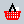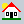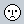CUMINCAD Papers